# Naive Bayes and Empirical Data¶

Often one has two empirically dervied datasets representative of two classes. Given a new data point, how can we decide which of our data sets the new point naturally belongs to?

One approach is classification using Bayes' Theorem, deriving empirical distributions from our sample data using kernel density estimates. This is essentially a Naive Bayes approach but we won't assume anything about the underlying empirical distributions (being e.g. normally distributed). That's another way of saying that this is a nonparametric method.

First let's generate and visualize some data.

In :
%matplotlib inline

In :
from matplotlib import pyplot as plt
import numpy as np
import pandas as pd
from scipy.stats import gaussian_kde
import seaborn as sns

from sklearn.neighbors.kde import KernelDensity


We'll use Beta distributions to generate two data sets.

In :
data1 = np.random.beta(2, 6, size=200)
data2 = np.random.beta(6, 2, size=300)

N1 = len(data1)
N2 = len(data2)

kde1 = gaussian_kde(data1)
kde2 = gaussian_kde(data2)

In :
plt.hist(data1, bins=20, alpha=0.5)
plt.hist(data2, bins=20, alpha=0.5)
plt.show()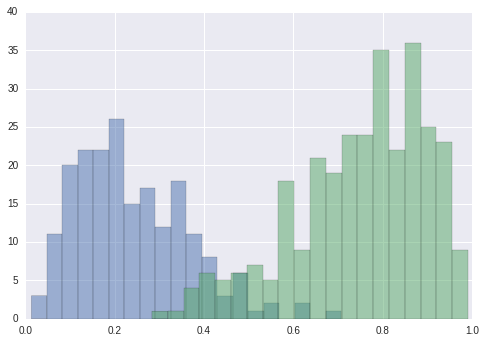In :
ax = sns.kdeplot(data1, shade=True, color="b")
plt.show()

/home/user/anaconda2/envs/py3/lib/python3.5/site-packages/statsmodels/nonparametric/kdetools.py:20: VisibleDeprecationWarning: using a non-integer number instead of an integer will result in an error in the future
y = X[:m/2+1] + np.r_[0,X[m/2+1:],0]*1j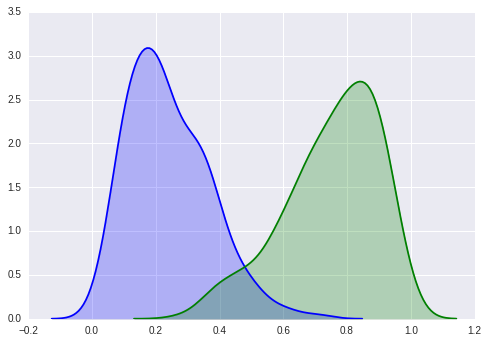Now let's use Bayes' Theorem to classify some test points.

$$Pr(\text{data}_1 \,| \,x) = \frac{Pr(x \, | \, \text{data}_1) Pr(\text{data}_1)}{Pr(x \,| \,\text{data}_1) Pr(\text{data}_1) + Pr(x \,| \,\text{data}_2) Pr(\text{data}_2)}$$
In :
def classify(x, kde1, kde2):
"""Computes the probabilities that the data point x
lies in dataset 1 versus dataset 2 using Bayes' Theorem."""
p1 = kde1.pdf(x)
p2 = kde2.pdf(x)
pd1 = p1 * N1 / (p1 * N1 + p2 * N2)
pd2 = p2 * N2 / (p1 * N1 + p2 * N2) # 1 - pd1
return (pd1, pd2)

In :
print(classify(0.2, kde1, kde2))
print(classify(0.5, kde1, kde2))
print(classify(0.8, kde1, kde2))

(0.99681750361056942, 0.003182496389430611)
(0.3297086021309012, 0.67029139786909875)
(0.001292606829725381, 0.99870739317027457)


We can visualize the probabilities of being in each class for every input value.

In :
xs = list(np.arange(0, 1, 0.01))
ps = []
qs = []
for x in xs:
p, q = classify(x, kde1, kde2)
ps.append(p)
qs.append(q)

plt.plot(xs, ps)
plt.plot(xs, qs)
plt.xlabel("Observed Value")
plt.ylabel("Predicted Class Probabilities")
plt.show()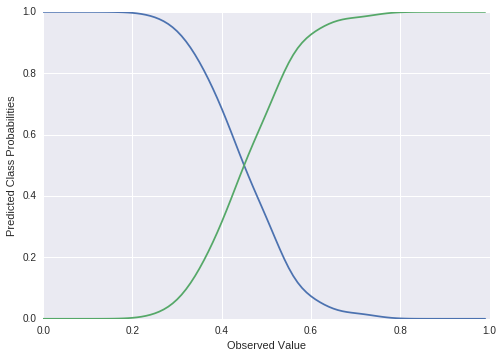Let's look at another example where the data sets are less separated.

In :
data1 = np.random.beta(3, 4, size=100)
data2 = np.random.beta(4, 3, size=100)

plt.show()

N1 = len(data1)
N2 = len(data2)

kde1 = gaussian_kde(data1)
kde2 = gaussian_kde(data2)

xs = list(np.arange(0, 1, 0.01))
ps = []
qs = []
for x in xs:
p, q = classify(x, kde1, kde2)
ps.append(p)
qs.append(q)

plt.plot(xs, ps)
plt.plot(xs, qs)
plt.xlabel("Observed Value")
plt.ylabel("Predicted Class Probabilities")
plt.show()

/home/user/anaconda2/envs/py3/lib/python3.5/site-packages/statsmodels/nonparametric/kdetools.py:20: VisibleDeprecationWarning: using a non-integer number instead of an integer will result in an error in the future
y = X[:m/2+1] + np.r_[0,X[m/2+1:],0]*1j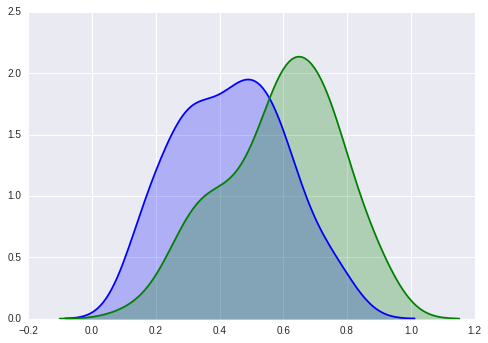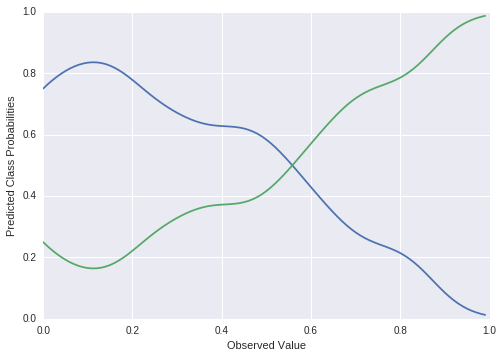## Classification Metrics¶

Now let's generate some new data and see how well our classifier performs.

In :
data1 = np.random.beta(3, 4, size=100)
data2 = np.random.beta(4, 3, size=100)

kde1 = gaussian_kde(data1)
kde2 = gaussian_kde(data2)

test_data1 = np.random.beta(3, 4, size=100)
test_data2 = np.random.beta(4, 3, size=100)

true_values =  * len(test_data1) +  * len(test_data2)

In :
predictions = []
for t in test_data1:
predictions.append(np.argmax(classify(t, kde1, kde2)))
for t in test_data2:
predictions.append(np.argmax(classify(t, kde1, kde2)))

In :
from sklearn.metrics import confusion_matrix, accuracy_score
print(confusion_matrix(true_values, predictions))
print("Accuracy:", accuracy_score(true_values, predictions))

[[60 40]
[28 72]]
Accuracy: 0.66


And the more separated data again.

In :
data1 = np.random.beta(3, 4, size=100)
data2 = np.random.beta(4, 3, size=100)

kde1 = gaussian_kde(data1)
kde2 = gaussian_kde(data2)

test_data1 = np.random.beta(2, 6, size=100)
test_data2 = np.random.beta(6, 2, size=100)

true_values =  * len(test_data1) +  * len(test_data2)

predictions = []
for t in test_data1:
predictions.append(np.argmax(classify(t, kde1, kde2)))
for t in test_data2:
predictions.append(np.argmax(classify(t, kde1, kde2)))

print(confusion_matrix(true_values, predictions))
print("Accuracy:", accuracy_score(true_values, predictions))

[[95  5]
[ 3 97]]
Accuracy: 0.96


Note that:

$$\frac{Pr(\text{data}_1 \,|\, x)}{Pr(\text{data}_2 \,|\, x)} = \frac{Pr(X \,|\, \text{data}_1)Pr(\text{data}_1)}{Pr(X\,|\, \text{data}_2)Pr(\text{data}_2)} = \frac{p_1(x)}{p_2(x)}$$

This means that the ratio of densities of the two distributions is the same as the classification ratio between the two classes. This is why it's harder to classify when the distributions are more overlapping (which should seem intuitively correct).

## Connection to Logistic Regression¶

If this seems similar to Logistic Regression then you've got good intuition! It turns out that if we assume the form of the true probability distributions is Gaussian, using Bayes Theorem is equivalent to Logistic Regression. Note that this turns our nonparametric method into a parametric method.

To see how this works, we have to rearrange Bayes' Theorem:

$$Pr(\text{data}_1 \,| \,x) = \frac{Pr(x \, | \, \text{data}_1) Pr(\text{data}_1)}{Pr(x \,| \,\text{data}_1) Pr(\text{data}_1) + Pr(x \,| \,\text{data}_2) Pr(\text{data}_2)}$$

as

$$Pr(\text{data}_1 \,| \,x) = \frac{1}{1 + \frac{Pr(x \,| \,\text{data}_2) Pr(\text{data}_2)}{Pr(x \,| \,\text{data}_1) Pr(\text{data}_1)} }$$

Then if we assume Gaussian distributions and slug through some algebra, we end up at something like

$$Pr(\text{data}_1 \,| \,x) = \frac{1}{1 + \exp{\left(w_0 + \sum_i{w_i X_i} \right)}} ,$$

aka Logistic Regression. There's a more complete derivation here.

## Digging deeper: Extending to Naive Bayes¶

Naive Bayes is a multidimensional form of this approach. It's called naive because we have to assume (conditional) independence of our covariates so that we can expand the joint probability distribution using the chain rule for probability. You can read more here.

In [ ]: